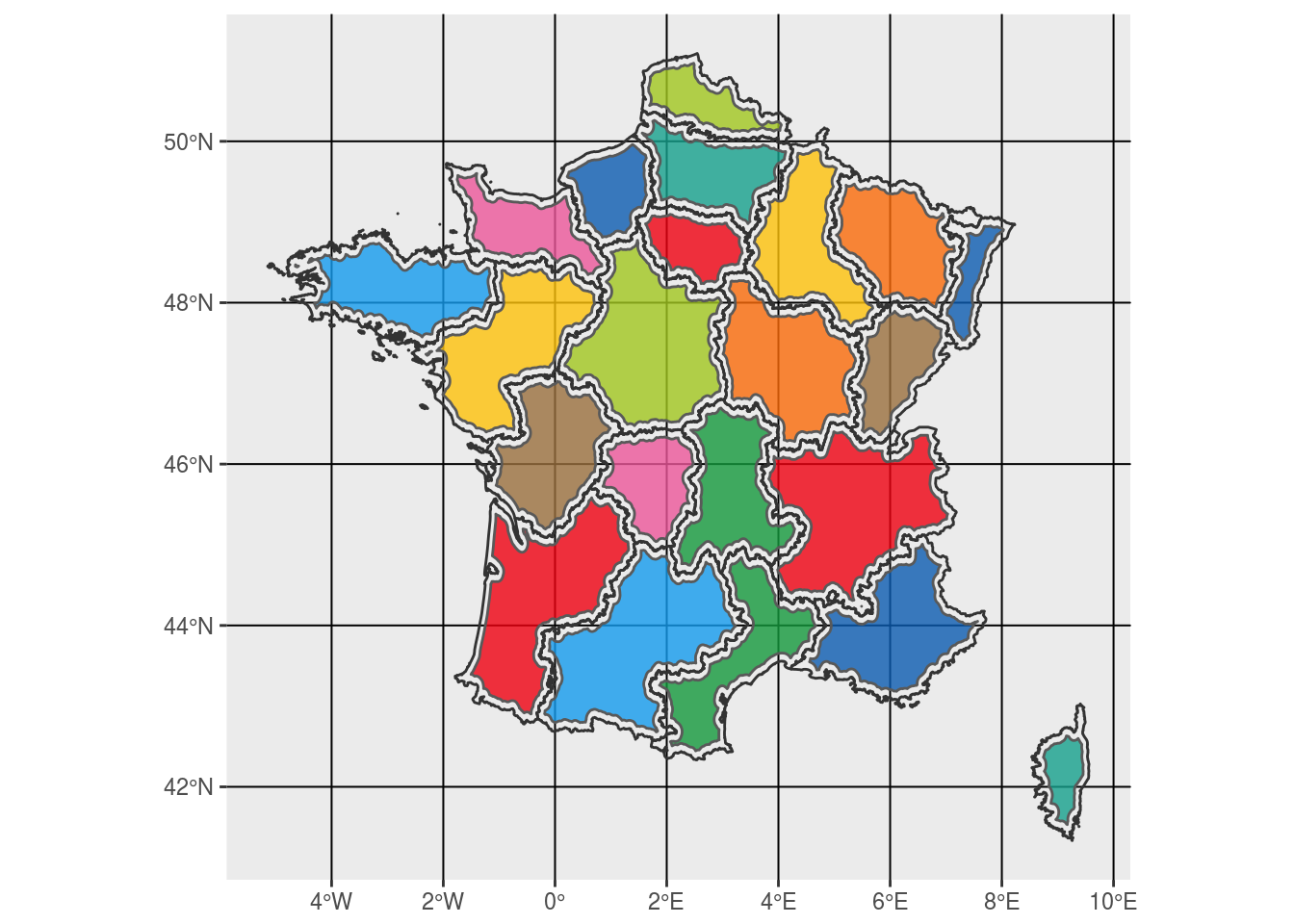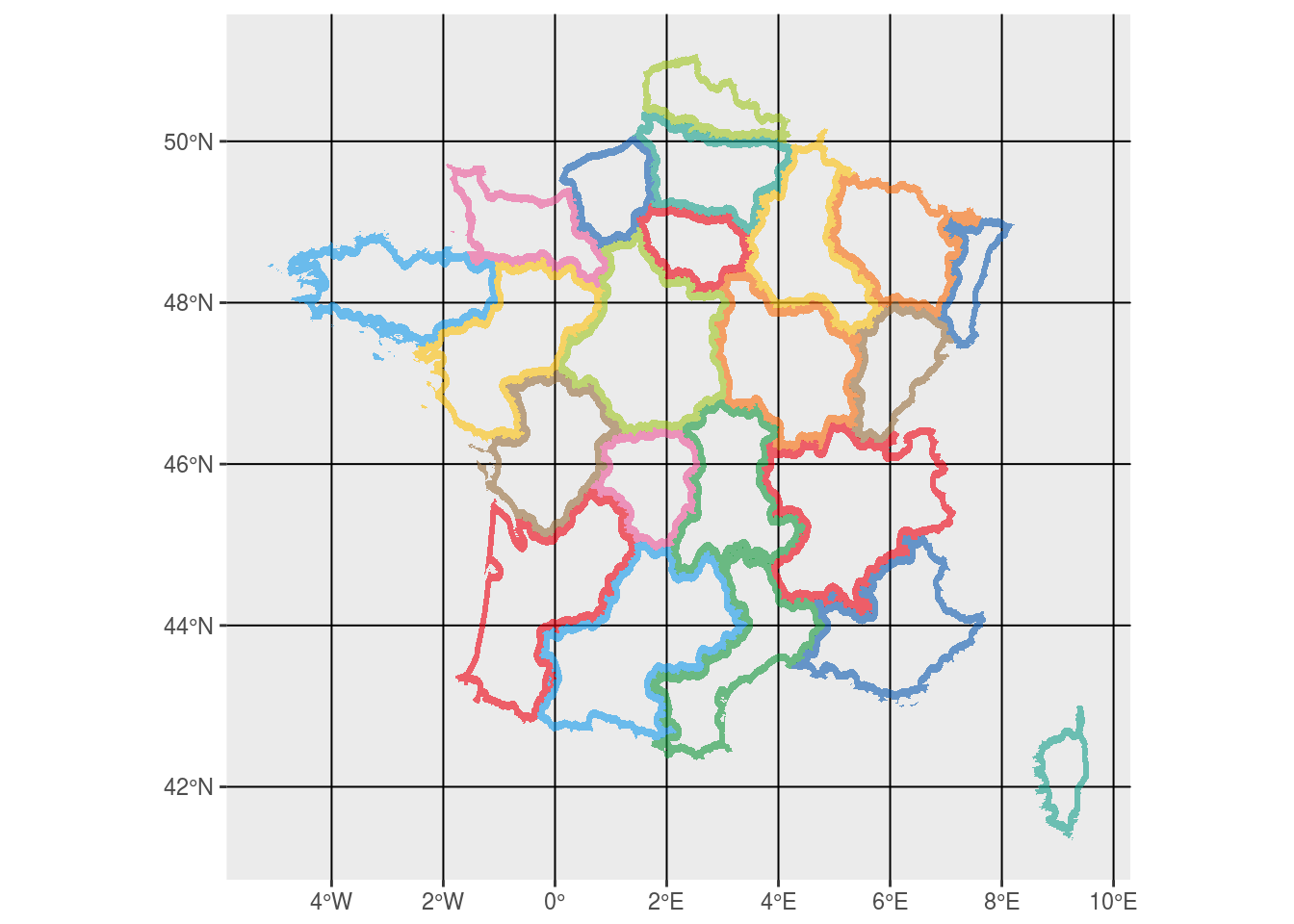# Polygons tint band with leaflet and simple feature (library sf)

## Add a tint band (aka shapeburst fill) in leaflet

Stackoverflow is again a source of inspiration. I found this question on tint bands in leaflet, not related to R originally but I though I could answer it with R easily. This is also a good one for me to play with this new simple feature library sf and use it with leaflet. My simple solution is to create a new multipolygon from the original one, but with holes inside, so that we only get a doughnut polygon for each area. As raised by my question on stackoverflow (again…), I recently remarked that it could be tricky to plot multipolygons with holes in ggplot2 with `SpatialPolygons` from library `sp`, which is another reason to use library `sf`.

## Get French regions data

Let’s use some French regions polygons and attribute one colour to each region. I like `piratepal` colours of library `yarrr`.

``````fra.sp <- getData('GADM', country = 'FRA', level = 1, path = extraWD)
fra.sf <- st_as_sf(fra.sp)

ggplot(fra.sf) +
geom_sf(aes(fill = NAME_1), alpha = 0.8) +
scale_fill_manual(values = rep(unique(yarrr::piratepal("basel")),
length.out = nrow(fra.sf))) +
guides(fill = FALSE)``````Figure 1: French regions colored using rstat and library `sf`

## Create future holes with buffer

We create the future holes with a smaller buffer area. Here, `st_buffer` returns an object without geometry type (`geometry type: GEOMETRY`), which prevents it to be plot (`Error in CPL_gdal_dimension(st_geometry(x), NA_if_empty)`). A workaround is to use `st_cast` after.

``````fra.sf.buf <- st_cast(st_buffer(fra.sf, dist = -0.1))

ggplot() +
geom_sf(data = fra.sf.buf, aes(fill = factor(NAME_1)), alpha = 0.8) +
geom_sf(data = fra.sf,
colour = "grey20", fill = "transparent",
size = 0.5) +
scale_fill_manual(values = rep(unique(yarrr::piratepal("basel")),
length.out = nrow(fra.sf))) +
guides(fill = FALSE)``````Figure 2: Polygon holes of future tint bands

## Create holes in the original polygons

To create the doughnuts using original and buffer polygons, I used `st_difference`. However as discussed with edzer in the `sf` github repository, I had to transform the buffer polygons into a Multipolygon of a unique Multipolygon using `st_combine`. Moreover, because the resulting Multipolygon object as no identified geometry, I had to pass it through `st_cast`.

``````# st_difference work if the mask is a unique multipolygon
fra.sf.buf.comb <- fra.sf.buf %>% st_combine() %>% st_sf()
fra.sf.doug <- st_difference(fra.sf, fra.sf.buf.comb) %>% st_cast()

ggplot() +
geom_sf(data = fra.sf.doug, aes(fill = factor(NAME_1)),
alpha = 0.6, colour = "transparent") +
# geom_sf(data = fra.sf, colour = "grey20") +
scale_fill_manual(values = rep(unique(yarrr::piratepal("basel")),
length.out = nrow(fra.sf.doug))) +
guides(fill = FALSE)``````Figure 3: Doughnuts style tint bands

## Output tint bands in leaflet

To allow for a quick view, polygons were simplified with `st_simplify`. You’ll see it if you zoom.

``````# Simplify geometry to get a lighter widget
# Separate "Rhône-Alpes", "Franche-Comté", "Bourgogne", "Bretagne" as it does not support to much simplification
fra.sf.doug.simple1 <- st_simplify(filter(fra.sf.doug, NAME_1 %in% c("Rhône-Alpes", "Franche-Comté")), dTolerance = 1e-4)
fra.sf.doug.simple2 <- st_simplify(filter(fra.sf.doug, NAME_1 %in% c("Bourgogne")), dTolerance = 1e-5)
fra.sf.doug.simple3 <- st_simplify(filter(fra.sf.doug, NAME_1 %in% c("Bretagne")), dTolerance = 1e-2)
fra.sf.doug.simple4 <- st_simplify(filter(fra.sf.doug, !NAME_1 %in% c("Rhône-Alpes", "Franche-Comté", "Bourgogne", "Bretagne")), dTolerance = 0.02)
fra.sf.doug.simple <- rbind(fra.sf.doug.simple1, fra.sf.doug.simple2, fra.sf.doug.simple3,  fra.sf.doug.simple4) %>% st_cast()
fra.sf.simple <- st_simplify(fra.sf, dTolerance = 0.02)``````

Now we can use it to produce a leaflet map with tinted bands having transparency and original polygons in black.

``````factpal <- colorFactor(rep(unique(yarrr::piratepal("basel")),
length.out = nrow(fra.sf.doug.simple)),
fra.sf.doug.simple\$NAME_1)

# leaflet widget
m <- leaflet() %>%
addPolygons(data = fra.sf.doug.simple, weight = 1, smoothFactor = 0.75,
opacity = 0, fillOpacity = 0.6,
color = "#000000",
fillColor = ~factpal(fra.sf.doug.simple\$NAME_1),
highlightOptions = highlightOptions(color = "white", weight = 2,
bringToFront = TRUE)) %>%
addPolygons(data = fra.sf.simple, weight = 1, smoothFactor = 0.75,
opacity = 1.0, fillOpacity = 0,
color = "#000000")``````

## The full rmarkdown script

If you want to reproduce these figures, the full rmarkdown script is available here on my github.

###### Citation:

``` Rochette Sébastien. (2017, Aug. 10). "Polygons tint band with leaflet and simple feature (library sf)". Retrieved from https://statnmap.com/2017-08-10-polygons-tint-band-with-leaflet-and-simple-feature-library-sf/. ```
``````@misc{Roche2017Polyg,